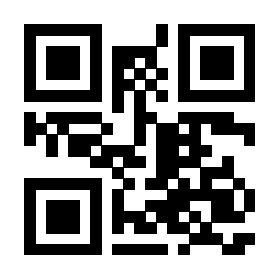# tblr环境插图的公式垂直居中问题？

## MWE

%图片是随便找的，表格公式和图片不搭，只是想研究排版效果

\documentclass{ctexart}
\usepackage{tabularray}
\usepackage{graphicx}
\begin{document}
\begin{tblr}{colspec={X[12em,c]X[10em,c]},rowspec={|Q[m]|Q[20em,m]|}}
图像 & 解集\\
\framebox{\raisebox{-2.5cm}{\includegraphics[width=0.4\textwidth]{tx.pdf}}}& $\{x \mid 0<x<4\}$\\
\end{tblr}\\[5mm]

\begin{tblr}{colspec={X[12em,c]X[10em,c]},rowspec={|Q[m]|Q[20em,m]|}}
图像 & 解集\\
\framebox{\includegraphics[width=0.4\textwidth]{tx.pdf}}& $\{x \mid 0<x<4\}$\\
\end{tblr}
\end{document}

### 编译效果0

1.1k
1 个回答

### 撰写答案